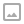## Bezier Curve Line Renderer

·   ·  ☕ 2 min read

Bezier Curves are the project of the day. Specifically we’re going to be looking at 3 point Bezier Curves and applying them to a Line Renderer in Unity.

Turns out that like shaders, bezier curves are one of those complex looking things that suggest a lot of math and theory. Turns out it’s just a lot of linear interpolation. Emphasis on the “lot”.

The curve is constructed by joining 3 points, point A, B and C. A and C represent the start and end of the line. B represents an additional control point that is used to adjust and control the curve. The line is created by calculating a number of points. First though another component the “ratio” or how far along the curve we are. This represents a percentage between 0 and 1 of where we are in our line.

The first step is two create two lines between points A → B and B → C. A point is selected between each line by using our ratio and Lerping between the two points on the line. This gives a new point somewhere along the line.

We can generate another line by connecting the new points calculated from both original lines.

To calculate the final point which we will place on our curve we again Lerp between the two calculated points using our given ratio.

By iterating over these points we can create a steady curve to render. Simple. The results are pretty neat to!

Discord: https://discord.gg/hU5Kq2uWRITTEN BY
Sam Wronski
Maker of things, currently helping build cloud things @ Google. World of Zero is a personal project disconnected from my professional work. Lets make something awesome together!